# Extract Numbers from a String in Excel (Using Formulas or VBA)

Watch Video – How to Extract Numbers from text String in Excel (Using Formula and VBA)

There is no inbuilt function in Excel to extract the numbers from a string in a cell (or vice versa –  remove the numeric part and extract the text part from an alphanumeric string).

However, this can be done using a cocktail of Excel functions or some simple VBA code.

Let me first show you what I am talking about.

Suppose you have a data set as shown below and you want to extract the numbers from the string (as shown below):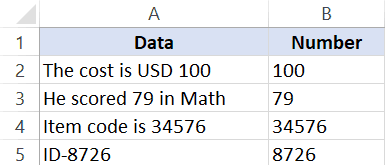The method you choose will also depend on the version of Excel you’re using:

• For versions prior to Excel 2016, you need to use slightly longer formulas
• For Excel 2016, you can use the newly introduced TEXTJOIN function
• VBA method can be used in all the versions of Excel

This Tutorial Covers:

## Extract Numbers from String in Excel (Formula for Excel 2016)

This formula will work only in Excel 2016 as it uses the newly introduced TEXTJOIN function.

Also, this formula can extract the numbers that are at the beginning, end or middle of the text string.

Note that the TEXTJOIN formula covered in this section would give you all the numeric characters together. For example, if the text is “The price of 10 tickets is USD 200”, it will give you 10200 as the result.

Suppose you have the dataset as shown below and you want to extract the numbers from the strings in each cell:

Below is the formula that will give you numeric part from a string in Excel.

`=TEXTJOIN("",TRUE,IFERROR((MID(A2,ROW(INDIRECT("1:"&LEN(A2))),1)*1),""))`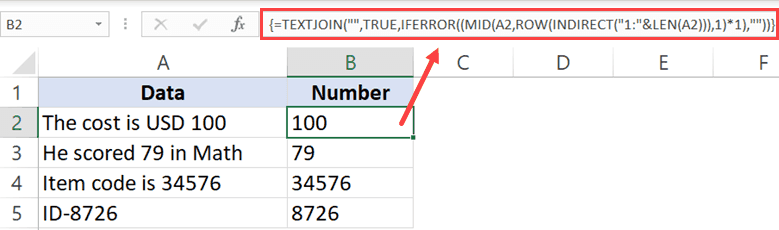This is an array formula, so you need to use ‘Control + Shift + Enter‘ instead of using Enter.

In case there are no numbers in the text string, this formula would return a blank (empty string).

How does this formula work?

Let me break this formula and try and explain how it works:

• ROW(INDIRECT(“1:”&LEN(A2))) – this part of the formula would give a series of numbers starting from one. The LEN function in the formula returns the total number of characters in the string. In the case of “The cost is USD 100”, it will return 19. The formulas would thus become ROW(INDIRECT(“1:19”). The ROW function will then return a series of numbers – {1;2;3;4;5;6;7;8;9;10;11;12;13;14;15;16;17;18;19}
• (MID(A2,ROW(INDIRECT(“1:”&LEN(A2))),1)*1) – This part of the formula would return an array of #VALUE! errors or numbers based on the string. All the text characters in the string become #VALUE! errors and all numerical values stay as-is. This happens as we have multiplied the MID function with 1.
• IFERROR((MID(A2,ROW(INDIRECT(“1:”&LEN(A2))),1)*1),””) – When IFERROR function is used, it would remove all the #VALUE! errors and only the numbers would remain. The output of this part would look like this – {“”;””;””;””;””;””;””;””;””;””;””;””;””;””;””;””;1;0;0}
• =TEXTJOIN(“”,TRUE,IFERROR((MID(A2,ROW(INDIRECT(“1:”&LEN(A2))),1)*1),””)) – The TEXTJOIN function now simply combines the string characters that remains (which are the numbers only) and ignores the empty string.
Pro Tip: If you want to check the output of a part of the formula, select the cell, press F2 to get into the edit mode, select the part of the formula for which you want the output and press F9. You will instantly see the result. And then remember to either press Control + Z or hit the Escape key. DO NOT hit the enter key.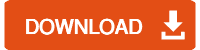You can also use the same logic to extract the text part from an alphanumeric string. Below is the formula that would get the text part from the string:

`=TEXTJOIN("",TRUE,IF(ISERROR(MID(A2,ROW(INDIRECT("1:"&LEN(A2))),1)*1),MID(A2,ROW(INDIRECT("1:"&LEN(A2))),1),""))`

A minor change in this formula is that IF function is used to check if the array we get from MID function are errors or not. If it’s an error, it keeps the value else it replaces it with a blank.

Then TEXTJOIN is used to combine all the text characters.

Caution: While this formula works great, it uses a volatile function (the INDIRECT function). This means that in case you use this with a huge dataset, it may take some time to give you the results. It’s best to create a backup before you use this formula in Excel.

## Extract Numbers from String in Excel (for Excel 2013/2010/2007)

If you have Excel 2013. 2010. or 2007, you can not use the TEXTJOIN formula, so you will have to use a complicated formula to get this done.

Suppose you have a dataset as shown below and you want to extract all the numbers in the string in each cell.The below formula will get this done:

`=IF(SUM(LEN(A2)-LEN(SUBSTITUTE(A2, {"0","1","2","3","4","5","6","7","8","9"}, "")))>0, SUMPRODUCT(MID(0&A2, LARGE(INDEX(ISNUMBER(--MID(A2,ROW(INDIRECT("\$1:\$"&LEN(A2))),1))* ROW(INDIRECT("\$1:\$"&LEN(A2))),0), ROW(INDIRECT("\$1:\$"&LEN(A2))))+1,1) * 10^ROW(INDIRECT("\$1:\$"&LEN(A2)))/10),"")`

In case there is no number in the text string, this formula would return blank (empty string).

Although this is an array formula, you don’t need to use ‘Control-Shift-Enter’ to use this. A simple enter works for this formula.

Credit to this formula goes to the amazing Mr. Excel forum.

Again, this formula will extract all the numbers in the string no matter the position. For example, if the text is “The price of 10 tickets is USD 200”, it will give you 10200 as the result.

Caution: While this formula works great, it uses a volatile function (the INDIRECT function). This means that in case you use this with a huge dataset, it may take some time to give you the results. It’s best to create a backup before you use this formula in Excel.

## Separate Text and Numbers in Excel Using VBA

If separating text and numbers (or extracting numbers from the text) is something you have to often, you can also use the VBA method.

All you need to do is use a simple VBA code to create a custom User Defined Function (UDF) in Excel, and then instead of using long and complicated formulas, use that VBA formula.

Let me show you how to create two formulas in VBA – one to extract numbers and one to extract text from a string.

### Extract Numbers from String in Excel (using VBA)

In this part, I will show you how to create the custom function to get only the numeric part from a string.

Below is the VBA code we will use to create this custom function:

```Function GetNumeric(CellRef As String)
Dim StringLength As Integer
StringLength = Len(CellRef)
For i = 1 To StringLength
If IsNumeric(Mid(CellRef, i, 1)) Then Result = Result & Mid(CellRef, i, 1)
Next i
GetNumeric = Result
End Function```

Here are the steps to create this function and then use it in the worksheet:

• Go to the Developer tab.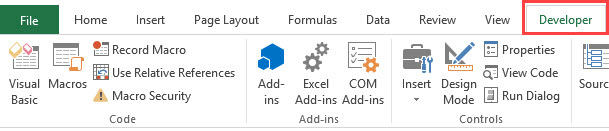• Click on Visual Basic (You can also use the keyboard shortcut ALT + F11)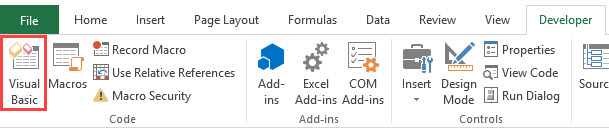• In the VB Editor backend that opens, right-click on any of the workbook objects.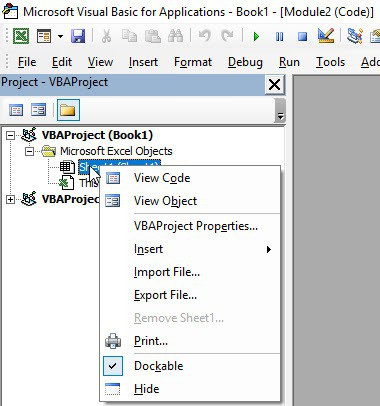• Go to Insert and click on Module. This will insert the module object for the workbook.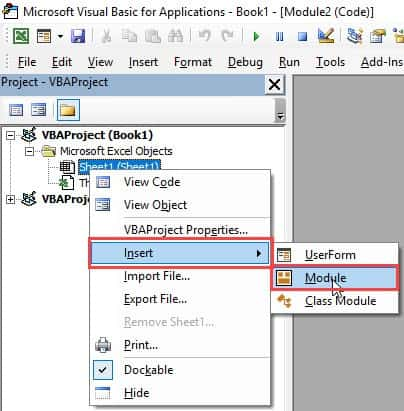• In the Module code window, copy and paste the VBA code mentioned above.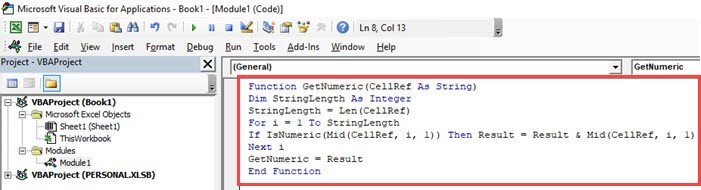• Close the VB Editor.

Now, you will be able to use the GetText function in the worksheet. Since we have done all the heavy lifting in the code itself, all you need to do is use the formula =GetNumeric(A2).

This will instantly give you only the numeric part of the string.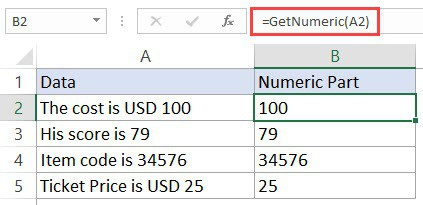Note that since the workbook now has VBA code in it, you need to save it with .xls or .xlsm extension.In case you have to use this formula often, you can also save this to your Personal Macro Workbook. This will allow you to use this custom formula in any of the Excel workbooks that you work with.

### Extract Text from a String in Excel (using VBA)

In this part, I will show you how to create the custom function to get only the text part from a string.

Below is the VBA code we will use to create this custom function:

```Function GetText(CellRef As String)
Dim StringLength As Integer
StringLength = Len(CellRef)
For i = 1 To StringLength
If Not (IsNumeric(Mid(CellRef, i, 1))) Then Result = Result & Mid(CellRef, i, 1)
Next i
GetText = Result
End Function```

Here are the steps to create this function and then use it in the worksheet:

• Go to the Developer tab.• Click on Visual Basic (You can also use the keyboard shortcut ALT + F11)• In the VB Editor backend that opens, right-click on any of the workbook objects.• Go to Insert and click on Module. This will insert the module object for the workbook.
• If you already have a module, double-click on it (no need to insert a new one if you already have it).• In the Module code window, copy and paste the VBA code mentioned above.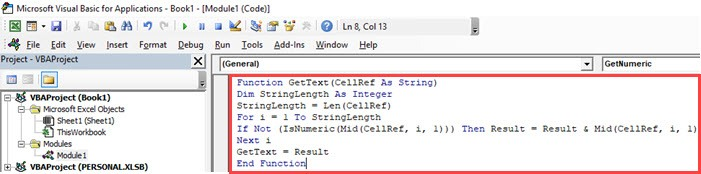• Close the VB Editor.

Now, you will be able to use the GetNumeric function in the worksheet. Since we have done all the heavy lifting in the code itself, all you need to do is use the formula =GetText(A2).

This will instantly give you only the numeric part of the string.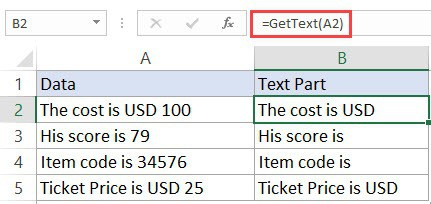Note that since the workbook now has VBA code in it, you need to save it with .xls or .xlsm extension.

In case you have to use this formula often, you can also save this to your Personal Macro Workbook. This will allow you to use this custom formula in any of the Excel workbooks that you work with.

In case you’re using Excel 2013 or prior versions and don’t have

You May Also Like the Following Excel Tutorials:FREE EXCEL BOOK

## Get 51 Excel Tips Ebook to skyrocket your productivity and get work done faster

• Flemings says:

Perfect Approaches well articulated.

• Daniel Abou-Chakra says:

it does not exist in 2016. i think it is n 360. i have 2016 and it is not there. had to use your VBA method which worked. so thank you

• Sayeed says:

Very useful. Really helped. Thanks.

• Rahul Sharma says:

Hi, I want to extract Alphanumeric number from the string for whole workbook having entries in thousands. And all the entries are of different type, please help. Entries are as shown below.
1). “2019-06-12 18:05:18– One RAX Card Faulty; card P Code: ROJ 119 2329/1 R18 Sr. No: (S)AE56819158. Reason: RED LED Continue on card, After Slot change, No change in status.”
from this entry i want A) “part code i.e “ROJ1192329/1” & B)Serial number i.e “AE56819158”
2). 2019-07-16 17:40:34– Two Nos of RU21 Card faulty at Fatehpur Kalan Node-B 3G BTS Both Card are checked replaced with working sector but card remain down with permanent RED LEG on card same has been conformed by JTO NAGAR Serial No:-1)AE56669151 , 2)CB47479036 Part Code:1)KRC118 16/3 R2A ,2) KRC 121 154/1 R3A
from this entry i want A) part code KRC121154/1 & B) Serial number AE56669151 & CB47479036

• Nikolina Kremmyda says:

how do we extract decimal values?

• William Nineteen says:

Hi Summit,

You might want to also consider the decimal symbol (period/dot “.” for North America, or comma “,” depending on the locale/country)

In most cases of a get numeric, you’ll want the decimal
I was processing US\$1,425K and I would get 1425 (the comma isn’t relevant)
But when I had US\$250.5K, I would get 12505. In this case, the desired number is 250.5 (the decimal is relevant)

So, I added Or Mid(CellRef, i, 1)=”.” for my purposes

Note that other countries would have US\$1.425,5K

• DIOCANE says:

DONT WORK FAGGOT

• Khalid Alzahrani says:

Dear, These cells have codes (? *) and (?) and (“Low Vol”) and numbers with code (67,306,444 *) how can I separate the numbers and use them for calculating. Unfortunately, function Getnumber not helping especially when all codes in the same column with more than 2 thousand cells.

Here the challenging

• rick boen says:

I’m using Excel 2016. The textjoin function is only available in 365. I put in the VBA formulas to no avail. Not only that, but now no functions or calculations will work on the spreadsheet. Please help.

• shishir sharma says:

Hi Sumit i watch all the published video of yours and find it interesting and useful….i need your small help today i wanted to convert numeric value in words without using macro if there is any formula for the same please help.

• Vikas G says:

UDF with a modification:-
———————————————————————-
Function GetNumeric(CellRef As String)
Dim StringLength As Integer
Dim Result ””””””””””””””””””””’
Result = “”
StringLength = Len(CellRef)
For i = 1 To StringLength
If IsNumeric(Mid(CellRef, i, 1)) Then Result = Result & Mid(CellRef, i, 1)
Next i
GetNumeric = Result * 1 ””””””””””””””””””””
End Function
—————————————————————————-
Function GetText(CellRef As String)
Dim StringLength As Integer
StringLength = Len(CellRef)
Dim Result As String ””””””””””””””””””””
Result = “”
For i = 1 To StringLength
If Not (IsNumeric(Mid(CellRef, i, 1))) Then Result = Result & Mid(CellRef, i, 1)
Next i
GetText = Result
End Function

• Franz says:

If the numeric part is always at the end, like in your examples, I think is more efficient the following formula to extract the numeric part: =VALUE(TRIM(MID(A2,FIND(“|”,SUBSTITUTE(A2,” “,”|”,LEN(A2)-LEN(SUBSTITUTE(A2,” “,””)))),9999999))) and the following one to extract just the text =TRIM(LEFT(A2,FIND(“|”,SUBSTITUTE(A2,” “,”|”,LEN(A2)-LEN(SUBSTITUTE(A2,” “,””))))))

• Thanks for sharing Franz.. This formula doesn’t work if there are no spaces. For example, if the text is “ABC123”, then it would return an error.

• Mohammed Masihuddin says:

Hey @Sumit, need your help on creating an Attendance tracker using formulas in Excel.